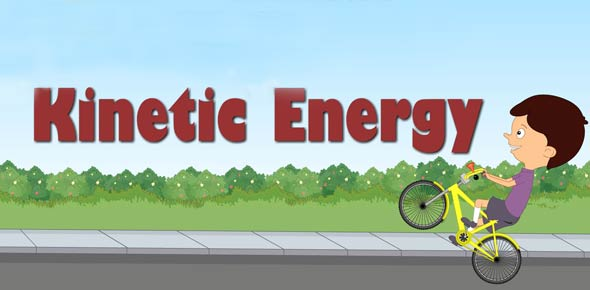Potential And Kinetic Energy

13 Questions | Total Attempts: 189Settings• 1.
The two main types of energy are...(bubble two bubbles)
• A.

Potential

• B.

Quiatonic

• C.

Hewlett

• D.

Kinetic

• E.

Ziatone

• 2.
________ Energy is the energy of motion...
• A.

Potential

• B.

Quiatonic

• C.

Hewlett

• D.

Kinetic

• E.

Ziatone

• 3.
________ Energy is the energy to have the potential to drop gain energy.
• A.

Potential

• B.

Quiatonic

• C.

Hewlett

• D.

Kinetic

• E.

Ziatone

• 4.
Which of the following is an example of kinetic energy...
• A.

None, you idiots! Because kinetic energy isn't real.

• B.

A guitar string singing

• C.

A dalmation

• D.

A rock sitting on top of a table

• E.

Nolan Ryan throwing a 100 mph fastball in a baseball game.

• 5.
Which is an example of potential energy?
• A.

None, you idiots! Because potential energy isn't real.

• B.

A guitar string singing

• C.

A dalmation

• D.

A rock sitting on a table

• E.

Randy Johnson throwing a 100 mph fastball in a baseball game.

• 6.
Which is an example of Quiatonic Energy...
• A.

None, you idiots! because quiatonic energy isn't real.

• B.

A guitar string singing

• C.

A dalmation

• D.

A rock sitting on a table

• E.

Cy Young throwing a 100mph pitch in a game.

• 7.
The formula for gravitational potential energy is....
• A.

Velocity x Mass

• B.

Mass x Gravity

• C.

Weight x Height

• D.

Gravity x Height

• E.

Mass x Distance

• 8.
The Formula for Power is...
• A.

Work Input/ Rapid Speed

• B.

Work Ammount/ Distance covered

• C.

Yellow/ Blue

• D.

Word Done / Time Interval

• E.

Force / Distance

• 9.
The Formula for work is...
• A.

Force x Distance

• B.

Work Input x Work Output

• C.

Velocity x Distance

• D.

Power x Force

• E.

Force x Horsepower

• 10.
The Formula to find the Kinetic Energy is....
• A.

1/2velocity x distance

• B.

1/2mass x Speed

• C.

Transformation x Work output

• D.

Mass ^2 x Distamnce

• E.

Veloctiy ^2 x Speed

• 11.
Work Output =
• A.

Blue

• B.

Green

• C.

True

• D.

Yellow

• E.

Work input

• 12.
The Law of conservation of energy is...
• A.

Energy can be created, but not destroyed. That is why there is such things as wimdmills and coal resources where so that we can make hybrids cars and stuff like that.

• B.

Energy cannot be created or destroyed; it may be transformed from one form to another, but the total amount of energy never changes.

• C.

Energy Can be created and destroyed. However, transformation cannot happen. It stays with the same type of energy it began with.

• D.

Energy cannot be created or destroyed; it may be transformed from quiatonic energy to hewlett and vice versa.

• E.

Energy Can be destroyed, but not created. Durring a transformation between one form to the other, energy is lost.

• 13.
Efficency's formula is....
• A.

Work Output/ Work input

• B.

Nolan/Ryan

• C.

Input Distance/ Output Distance

• D.

Rodger/ Clemens

• E.

Usefull Work Output / Total Work Input

Related TopicsBack to top